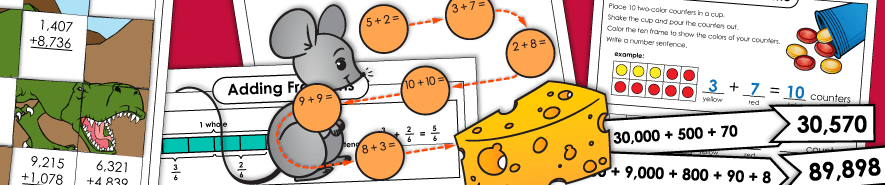# 基本的补充事实## 基本添加

（示例：3+2 = 5，1+3 = 4）

（示例：9+8 = 17，8+6 = 14，7+6 = 13）

## 基本的添加概念和策略

（示例：5+5 = 10，6+4 = 10，7+3 = 10）

（示例：2+2 = 4，4+4 = 8，8+8 = 16，10+10 = 20）

（示例：3+4 = 7，5+6 = 11，7+8 = 15，8+9 = 17）

（正数和负数）

2位添加（无重组）

2位添加（与重组）

3位添加

4位添加

5位添加

## 增加小数，金钱和分数